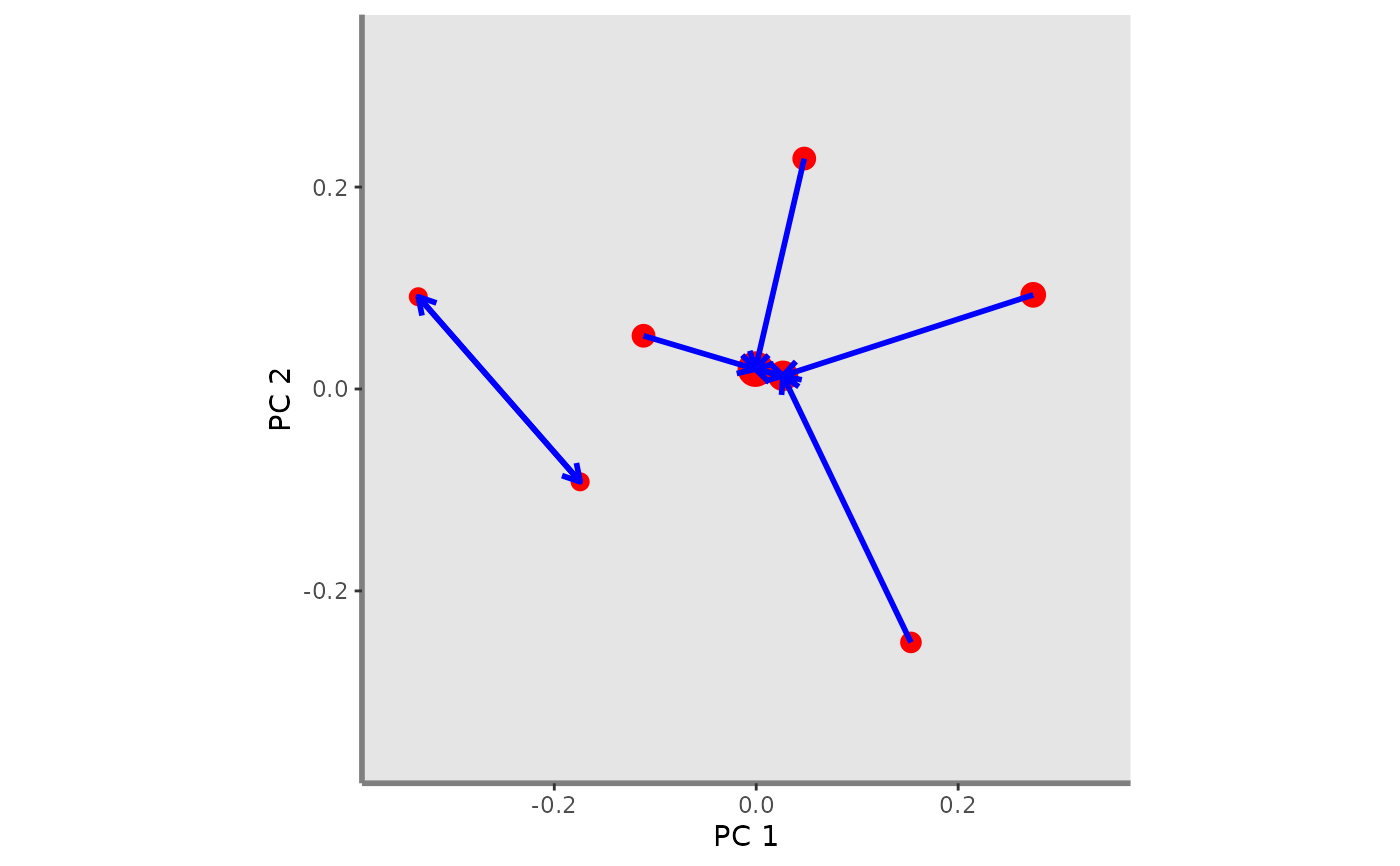This function plots FNND index for a given pair of functional axes and for one or several assemblages

fnnd.plot(
ggplot_bg,
asb_sp_coord2D,
asb_sp_relatw,
asb_nn_asb,
plot_sp = TRUE,
shape_sp,
color_sp,
fill_sp,
color_segment,
width_segment,
linetype_segment
)

## Arguments

ggplot_bg

a ggplot object of the plot background retrieved through the background.plot function.

asb_sp_coord2D

a list of matrix (ncol = 2) with coordinates of species present in each assemblage for a given pair of functional axes

asb_sp_relatw

a list of vector gathering species relative weight in each assemblage. It can be retrieved through the alpha.fd.multidim.

asb_nn_asb

a list gathering for each species of a studied assemblage its nearest neighbour(s) in the assemblage.

plot_sp

a logical value indicating whether species of each assemblage should be plotted or not. Default: plot_sp = TRUE

shape_sp

a numeric value referring to the shape used to plot species belonging to the studied assemblage.

color_sp

a R color name or an hexadecimal code referring to the color of species if one assemblage to plot or a vector of R color names or hexadecimal codes if several assemblages to plot. If more than one assemblage to plot, the vector should be formatted as: c(asb1 = "firstRcolorname", asb2 = "secondRcolorname", ...).

fill_sp

a R color name or an hexadecimal code referring to the color of species symbol filling (if shape_sp > 20) if one assemblage to plot or a vector of R color names or hexadecimal codes if several assemblages to plot. If more than one assemblage to plot, the vector should be formatted as: c(asb1 = "firstRcolorname", asb2 = "secondRcolorname", ...).

color_segment

a R color name or an hexadecimal code referring to the color of of the segment linking nearest neighbors in a given assemblage or a vector of R color names or hexadecimal codes if several assemblages to plot. If more than one assemblage to plot, the vector should be formatted as: c(asb1 = "firstRcolorname", asb2 = "secondRcolorname", ...).

width_segment

a numeric value referring to the size of the segment linking nearest neighbors in a given assemblage or a vector of numeric values if several assemblages to plot. If more than one assemblage to plot, the vector should be formatted as: c(asb1 = "firstRwidth", asb2 = "secondRwidth", ...).

linetype_segment

a character string referring to the linetype used to link nearest neighbors in the studied assemblages or a vector of character strings if several assemblages to plot. If more than one assemblage to plot, the vector should be formatted as: c(asb1 = "firstRlinetype", asb2 = "secondRlinetype", ...).

## Value

A ggplot object with FNND index.

## Author

Camille Magneville and Sebastien Villeger

## Examples

# Load Species*Traits dataframe:
data("fruits_traits", package = "mFD")

data("fruits_traits_cat", package = "mFD")

# Compute functional distance
sp_dist_fruits <- mFD::funct.dist(sp_tr         = fruits_traits,
tr_cat        = fruits_traits_cat,
metric        = "gower",
scale_euclid  = "scale_center",
ordinal_var   = "classic",
weight_type   = "equal",
stop_if_NA    = TRUE)
#>  "Running w.type=equal on groups=c(Size)"
#>  "Running w.type=equal on groups=c(Plant)"
#>  "Running w.type=equal on groups=c(Climate)"
#>  "Running w.type=equal on groups=c(Seed)"
#>  "Running w.type=equal on groups=c(Sugar)"
#>  "Running w.type=equal on groups=c(Use,Use,Use)"

# Compute functional spaces quality to retrieve species coordinates matrix:
fspaces_quality_fruits <- mFD::quality.fspaces(sp_dist = sp_dist_fruits,
maxdim_pcoa         = 10,
deviation_weighting = "absolute",
fdist_scaling       = FALSE,
fdendro             = "average")

# Retrieve species coordinates matrix:
sp_faxes_coord_fruits <- fspaces_quality_fruits$details_fspaces$sp_pc_coord

# Set faxes limits:
# set range of axes if c(NA, NA):
range_sp_coord_fruits  <- range(sp_faxes_coord_fruits)
range_faxes_lim <- range_sp_coord_fruits +
c(-1, 1)*(range_sp_coord_fruits -
range_sp_coord_fruits) * 0.05

# Retrieve the background plot:
ggplot_bg_fruits <- mFD::background.plot(
range_faxes = range_faxes_lim,
faxes_nm    = c("PC 1", "PC 2"),
color_bg    = "grey90")

# Retrieve the matrix of species coordinates for "basket_1" and PC1 and PC2
sp_faxes_coord = sp_faxes_coord_fruits,
fruits_asb_sp_coord_b1 <- sp_filter$species coordinates fruits_asb_sp_coord2D_b1 <- fruits_asb_sp_coord_b1[, c("PC1", "PC2")] # Use alpha.fd.multidim() function to get inputs to plot FIde: alpha_fd_indices_fruits <- mFD::alpha.fd.multidim( sp_faxes_coord = sp_faxes_coord_fruits[, c("PC1", "PC2", "PC3", "PC4")], asb_sp_w = baskets_fruits_weights, ind_vect = c("fnnd"), scaling = TRUE, check_input = TRUE, details_returned = TRUE) #> basket_1 done 10% #> basket_2 done 20% #> basket_3 done 30% #> basket_4 done 40% #> basket_5 done 50% #> basket_6 done 60% #> basket_7 done 70% #> basket_8 done 80% #> basket_9 done 90% #> basket_10 done 100% # Retrieve nearest neighbor(s) names and relative weights ... # ... through alpha.fd.multidim outputs: fruits_asb_nn_asb_b1 <- alpha_fd_indices_fruits$details$asb_nm_nn_asb["basket_1"] fruits_asb_sp_relatw_b1 <- alpha_fd_indices_fruits$details\$asb_sp_relatw["basket_1", ]

# Retrieve FNND plot:
fnnd_plot <- fnnd.plot(ggplot_bg = ggplot_bg_fruits,